Open in App
Not now

# Anti-aliased Line | Xiaolin Wu’s algorithm

• Difficulty Level : Medium
• Last Updated : 07 Jan, 2022

Anti-Aliased Line Drawing
Below is the image showing line drawn with Bresenham’s line algorithm (left) and Xiaolin Wu’s line algorithm (right) which smooths the line. Which one looks better to you ?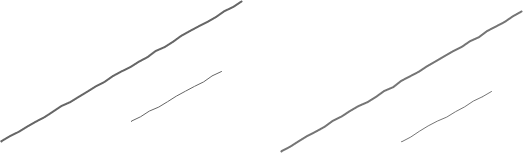Anti Aliasing concept
Suppose we want to draw a line from point(1 , 1) to point(8 , 4) with rectangular edges. The ideal line would be the one shown in figure A . Since I want to display it on screen I cannot use that. Line needs to go through a process called Rasterization which would determine color of individual pixels. Several algorithms can be used like Bresenham’s Line Algorithm , Digital Differential Analyzer , Xiaolin Wu’s line algorithm , Gupta-Sproull algorithm . Later two perform anti-aliasing or line smoothing.
The result produced by first two algorithm is show in figure B.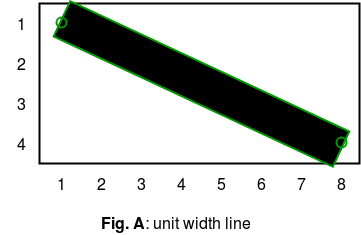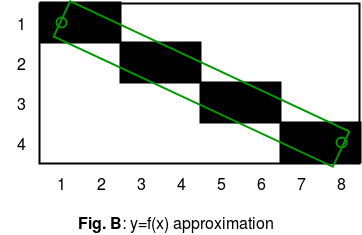There are few problems in Line( figure B ).
1. Pixel (4,2) has less coverage than Pixel (3,2), yet they’re both drawn fully black.
2. Pixel (2,2) has almost as much coverage as (4,2), and yet it’s drawn fully white.
To overcome these drawbacks and produce a much smoother looking line we use Xiaolin Wu’s line algorithm

Xiaolin Wu’s line algorithm
Consider the figure shown below which is drawn using Bresenham’s Line Generation Algorithm . Take a segment and its initial coordinate x. By the X in the loop is added 1 towards the end of the segment. At each step, the error is calculated – the distance between the actual y-coordinate at that location and the nearest grid cell. If the error does not exceed half the height of the cell, it is filled. That’s the whole algorithm.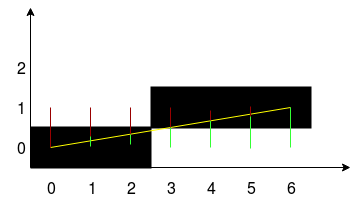We will modify this algorithm so that it can produce an anti-aliased line .
Xiaolin Wu’s line algorithm is characterized by the fact that at each step of the calculation is carried out for the two closest to the line of pixels, and they are colored with different intensity, depending on the distance. Current intersection middle pixel intensity gives 100% if the pixel is within 0.9 pixel, the intensity will be 10%. In other words, one hundred percent of the intensity is divided between the pixels which limit vector line on both sides.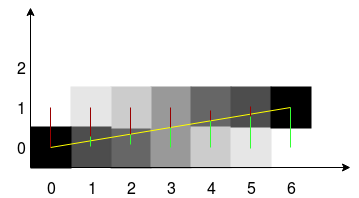In the picture the red and green color shows the distance to the two adjacent pixels. To calculate the error, you can use floating point and take the error value of the fractional part.

NOTE:The following implementation uses SDL library to draw pixels on screen . If you are on debian system like ubuntu just run following command to install SDL library.

```sudo apt-get install libsdl2-dev
```

To build use

```gcc filename.c -lSDL2
```

## Recommended: Please try your approach on {IDE} first, before moving on to the solution.

Note:If the projection of the segment on the x-axis is less than the projection on the y-axis, or the beginning and end of the segment are swapped, then the algorithm will not work. To avoid this, you need to check the direction of the vector and its slope, and then swap the coordinates of the line , ultimately to reduce everything to some one or at least two cases.
Following algorithm assumes that only integer co-ordinates will be given as inputs since pixel value cannot be floating point.

 `// C program to implement Xiaolin Wu's line drawing``// algorithm.``// We must install SDL library using above steps``// to run this program``#include`` ` `// SDL stuff``SDL_Window* pWindow = 0;``SDL_Renderer* pRenderer = 0;`` ` `// swaps two numbers``void` `swap(``int``* a , ``int``*b)``{``    ``int` `temp = *a;``    ``*a = *b;``    ``*b = temp;``}`` ` `// returns absolute value of number``float` `absolute(``float` `x )``{``    ``if` `(x < 0) ``return` `-x;``    ``else` `return` `x;``}`` ` `//returns integer part of a floating point number``int` `iPartOfNumber(``float` `x)``{``    ``return` `(``int``)x;``}`` ` `//rounds off a number``int` `roundNumber(``float` `x)``{``    ``return` `iPartOfNumber(x + 0.5) ;``}`` ` `//returns fractional part of a number``float` `fPartOfNumber(``float` `x)``{``    ``if` `(x>0) ``return` `x - iPartOfNumber(x);``    ``else` `return` `x - (iPartOfNumber(x)+1);`` ` `}`` ` `//returns 1 - fractional part of number``float` `rfPartOfNumber(``float` `x)``{``    ``return` `1 - fPartOfNumber(x);``}`` ` `// draws a pixel on screen of given brightness``// 0<=brightness<=1. We can use your own library``// to draw on screen``void` `drawPixel( ``int` `x , ``int` `y , ``float` `brightness)``{``    ``int` `c = 255*brightness;``    ``SDL_SetRenderDrawColor(pRenderer, c, c, c, 255);``    ``SDL_RenderDrawPoint(pRenderer, x, y);``}`` ` `void` `drawAALine(``int` `x0 , ``int` `y0 , ``int` `x1 , ``int` `y1)``{``    ``int` `steep = absolute(y1 - y0) > absolute(x1 - x0) ;`` ` `    ``// swap the co-ordinates if slope > 1 or we``    ``// draw backwards``    ``if` `(steep)``    ``{``        ``swap(&x0 , &y0);``        ``swap(&x1 , &y1);``    ``}``    ``if` `(x0 > x1)``    ``{``        ``swap(&x0 ,&x1);``        ``swap(&y0 ,&y1);``    ``}`` ` `    ``//compute the slope``    ``float` `dx = x1-x0;``    ``float` `dy = y1-y0;``    ``float` `gradient = dy/dx;``    ``if` `(dx == 0.0)``        ``gradient = 1;`` ` `    ``int` `xpxl1 = x0;``    ``int` `xpxl2 = x1;``    ``float` `intersectY = y0;`` ` `    ``// main loop``    ``if` `(steep)``    ``{``        ``int` `x;``        ``for` `(x = xpxl1 ; x <=xpxl2 ; x++)``        ``{``            ``// pixel coverage is determined by fractional``            ``// part of y co-ordinate``            ``drawPixel(iPartOfNumber(intersectY), x,``                        ``rfPartOfNumber(intersectY));``            ``drawPixel(iPartOfNumber(intersectY)-1, x,``                        ``fPartOfNumber(intersectY));``            ``intersectY += gradient;``        ``}``    ``}``    ``else``    ``{``        ``int` `x;``        ``for` `(x = xpxl1 ; x <=xpxl2 ; x++)``        ``{``            ``// pixel coverage is determined by fractional``            ``// part of y co-ordinate``            ``drawPixel(x, iPartOfNumber(intersectY),``                        ``rfPartOfNumber(intersectY));``            ``drawPixel(x, iPartOfNumber(intersectY)-1,``                          ``fPartOfNumber(intersectY));``            ``intersectY += gradient;``        ``}``    ``}`` ` `}`` ` `// Driver code``int` `main(``int` `argc, ``char``* args[])``{`` ` `    ``SDL_Event event;`` ` `    ``// initialize SDL``    ``if` `(SDL_Init(SDL_INIT_EVERYTHING) >= 0)``    ``{``        ``// if succeeded create our window``        ``pWindow = SDL_CreateWindow(``"Anti-Aliased Line "``,``                     ``SDL_WINDOWPOS_CENTERED, SDL_WINDOWPOS_CENTERED,``                                                          ``640, 480,``                                                  ``SDL_WINDOW_SHOWN);`` ` `        ``// if the window creation succeeded create our renderer``        ``if` `(pWindow != 0)``            ``pRenderer = SDL_CreateRenderer(pWindow, -1, 0);``    ``}``    ``else``        ``return` `1; ``// sdl could not initialize`` ` `    ``while` `(1)``    ``{``        ``if` `(SDL_PollEvent(&event) && event.type == SDL_QUIT)``            ``break``;`` ` `        ``// Sets background color to white``        ``SDL_SetRenderDrawColor(pRenderer, 255, 255, 255, 255);``        ``SDL_RenderClear(pRenderer);`` ` `        ``// draws a black AALine``        ``drawAALine(80 , 200 , 550, 150);`` ` `        ``// show the window``        ``SDL_RenderPresent(pRenderer);``    ``}`` ` `    ``// clean up SDL``    ``SDL_Quit();``    ``return` `0;``}`

Output:

```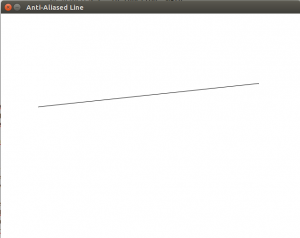```

References:
https://courses.engr.illinois.edu/ece390/archive/archive-f2000/mp/mp4/anti.html
https://unionassets.com/blog/algorithm-brezenhema-and-wu-s-line-299
https://en.wikipedia.org/wiki/Xiaolin_Wu%27s_line_algorithm
This article is contributed by Lokesh Sharma. If you like GeeksforGeeks and would like to contribute, you can also write an article using write.geeksforgeeks.org or mail your article to review-team@geeksforgeeks.org. See your article appearing on the GeeksforGeeks main page and help other Geeks.